# C program to check if a given string is a palindrome

In this article, you will learn how to check whether a given string is a palindrome or not. Here is the list of programs available here:

But before going through these programs, let's talk about the meaning of a palindrome string.

### What is a palindrome string?

Palindromes are strings in which all of the opposite characters are the same. And if any of the opposite characters mismatch, then the string is not a palindrome. For example:

• codoc is a palindrome string because:
• The first character (c) is equal to the last character (c).
• The second character (o) is the same as the second-last character (o).
• The third character (d) is equal to the third character from the last (d).
• codssdoc is also a palindrome string because:
• The first character (c) is equal to the last character (c).
• The second character (o) is equal to the second-last character (o).
• The third character (d) is equal to the third-last character (d).
• The fourth character (s) is equal to the fourth-last character (s).

In other words, you can say that if the reverse of a string is equal to its original, then that string can be called a palindrome string.

## Palindrome String Program in C

The question is: write a program in C to enter any string at run-time and check whether it is a palindrome or not. The answer to this question is:

```#include<stdio.h>
#include<conio.h>
#include<string.h>
int main()
{
char str;
int i, j, len, chk=0;
printf("Enter any String (word): ");
scanf("%s", str);
len = strlen(str);
for(i=0, j=(len-1); i<=(len-1); i++, j--)
{
if(str[i] != str[j])
{
chk=1;
break;
}
}
if(chk==1)
printf("\nIt's not a Palindrome String");
else
printf("\nIt's a Palindrome String");
getch();
return 0;
}```

The above program is written in the CodeBlocks IDE. Here is the output after building and running. This is the first screenshot of the first sample run: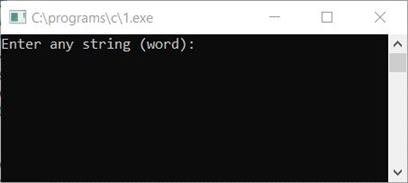And here is the second screenshot of the first sample run: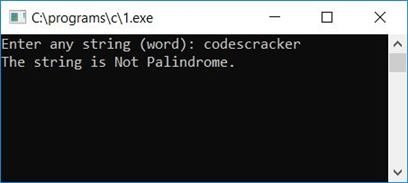As the reverse of codescracker is rekcarcsedoc, which is not equal to the original string, that is codescracker, therefore the string is not a palindrome.

Here is the first screenshot of the second sample run: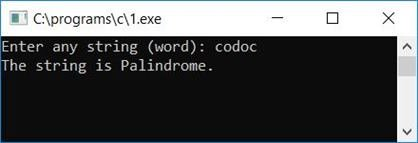Here in this case, the reverse of codoc is codoc, which is the same as the original string, that is, codoc, therefore, the string is a palindrome.

#### Program Explained

• First, we have to count the length of the string using the strlen() function. For example, let's suppose the length of the string is 6.
• Create a loop to compare the first character of the string to the last, and then the second to the second last, and so on. Therefore, the first character is compared to the sixth character, the second to the fifth, and the third to the fourth. Because indexing begins at 0, we must subtract one from all. That is, 0th to length-1.
• If any of the above comparisons go wrong, or if any character mismatches, then we have to initialize any number, say 1, to any character, say chk, and skip the remaining code of the loop using the break statement.
• After exiting from the loop, whether using a break statement or after running the whole loop, We have to check whether the variable chk holds its original value, that is, 0 or not. If it holds its original value, then none of the opposite characters mismatch (meaning it is a palindrome), and if it doesn't hold its original value, then any of the opposite characters mismatch (meaning it is not a palindrome).
• The string is not a palindrome if there is a mismatch; otherwise, the string is a palindrome.

Here is another sample run for a palindrome string: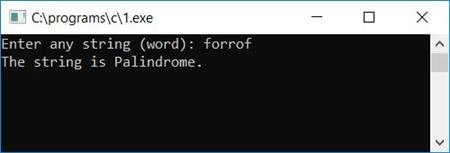And here is one more sample run, not a palindrome string: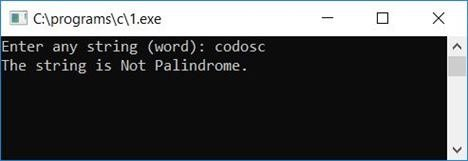### Check palindrome strings in C without using the string function

Here is another program that will not use any string functions. As you can see from the previous program, the string function strlen() is used to find the length of the entered string. But here, this program will calculate the length of a string without using any library functions.

```#include<stdio.h>
#include<conio.h>
int main()
{
char str;
int i, j, len, chk=0;
printf("Enter any String (word): ");
scanf("%s", str);
len = 0;
while(str[len]!='\0')
len++;
for(i=0, j=(len-1); i<=(len-1); i++, j--)
{
if(str[i] != str[j])
{
chk=1;
break;
}
}
if(chk==1)
printf("\nIt's not a Palindrome String");
else
printf("\nIt's a Palindrome String");
getch();
return 0;
}```

The code:

```len=0;
while(str[len]!='\0')
len++;```

is used to find the length of a string. For example, if the user enters codes as input, 0 is initialized to len before entering the while loop. The '\0' is known as a null terminated character. At the end of every string, a null-terminated character gets automatically initialized. So the dry run of the above code works like this:

• For the first run, str[len] or str or c is not equal to '\0'. Therefore, len++ gets executed, so the value of len gets incremented and becomes 1.
• For the second run, str[len] or str or o is not equal to '\0'. Therefore, len++ gets executed, so the value of len gets incremented and becomes 2.
• For the third run, str[len] or str or d is not equal to '\0'. Therefore, len++ gets executed, so the value of len gets incremented and becomes 3.
• For the sixth run, str[len] or str or '\0' is equal to '\0'. Therefore, the condition evaluates to be false, so the loop gets terminated, and we have a variable, len, that holds the length value of the string.

## Check palindrome strings in C using the function

This is the last program to check whether a given string is a palindrome or not using a user-defined function.

```#include<stdio.h>
#include<conio.h>
int checkPalindromeStr(char []);
int main()
{
char str;
int chk;
printf("Enter any String (word): ");
scanf("%s", str);
chk = checkPalindromeStr(str);
if(chk==1)
printf("\nIt's not a Palindrome String");
else
printf("\nIt's a Palindrome String");
getch();
return 0;
}
int checkPalindromeStr(char str[])
{
int i, j, len;
len = 0;
while(str[len]!='\0')
len++;
for(i=0, j=(len-1); i<=(len-1); i++, j--)
{
if(str[i] != str[j])
return 1;
}
return 0;
}```

This program will produce the same output as the first program given earlier in this article.

C Quiz

« Previous Program Next Program »